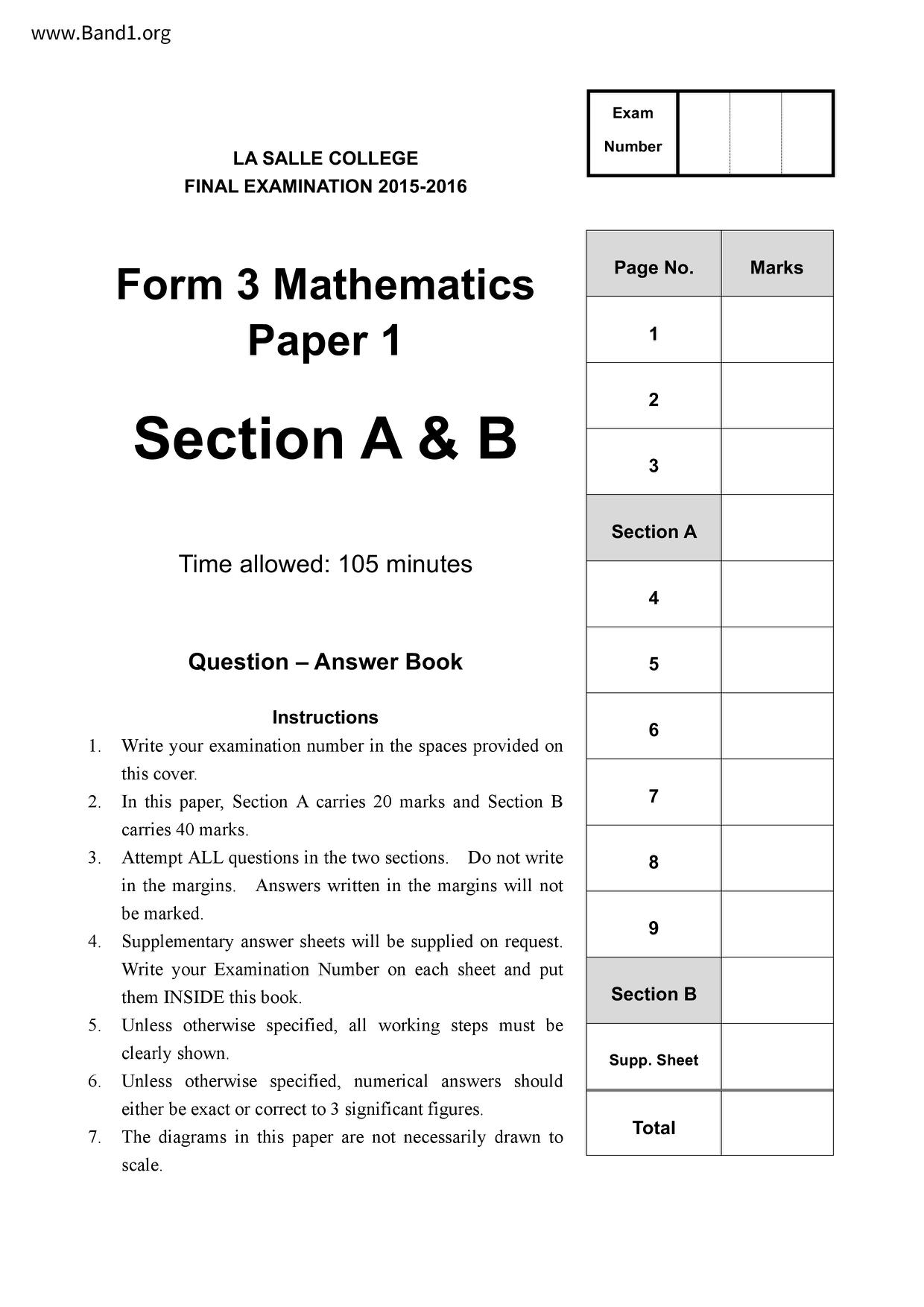# 中三 數學試卷 (F3 Maths Past Paper)

6630

pdf

11

maths 1516_F3_FINAL_Paper_1_AB

▼ 圖片只作預覽, 如欲下載整份卷, 請按「免費成為會員」 ▼▲ 圖片只作預覽, 如欲下載整份卷, 請按「免費成為會員」 ▲

## 中三數學試卷 PDF 下載

LA SALLE COLLEGE
FINAL EXAMINATION 2015-2016
Form 3 Mathematics
Section A & B
Time allowed: 105 minutes
Instructions
1. Write your examination number in the spaces provided on
this cover.
In this paper, Section A carries 20 marks and Section B
carries 40 marks.
3. Attempt ALL questions in the two sections. Do not write
in the margins. Answers written in the margins will not
4. Supplementary answer sheets will be supplied on request.
Write your Examination Number on each sheet and put
them INSIDE this book.
5. Unless otherwise specified, all working steps must be
clearly shown.
6. Unless otherwise specified, numerical answers should
either be exact or correct to 3 significant figures.
7. The diagrams in this paper are not necessarily drawn to
Supp. Sheet
13. In the figure, GC // FD. E is a point on FD such that
AE and BE cut BF and AD at G and C respectively. If
G is mid-point of AE, C is mid-point of EB and DE =
cm, find x, y and z.
Form 3 Maths - Final Exam 2015-2016
Answers written in the margins will not be marked
Form 3 Maths - Final Exam 2015-2016
- End of Section A and B-
Answers written in the margins will not be marked
Section A [20 marks]
1. Simplify
(a²b-³)-³
and express the answer with positive indices.
2. Make a the subject of the formula xy - ay = c(ax - y²).
Form 3 Maths - Final Exam 2015-2016
Answers written in the margins will not be marked
a) Factorize a³ +27.
b) Factorize a³ - 7a+6.
Form 3 Maths - Final Exam 2015-2016
Answers written in the margins will not be marked
5. a) Solve the inequality 2x+5+x=5>3x+4+1
b) Write the smallest integer satisfying the above inequality.
6. Mr. Chan deposits \$400 000 at a bank at an interest rate of 8% p.a. compounded quarterly.
a) Find the amount he will get after 21 months.
b) Find the interest he will get.
Form 3 Maths - Final Exam 2015-2016
Answers written in the margins will not be marked
Section B [40 marks]
7. The table below shows the salaries tax rate for the year 2016/17:
Net chargeable income
On the first \$40 000
On the next \$40 000
On the next \$40 000
Mr. Chan's net chargeable income is \$60000 and his salaries tax is \$2000. Find the
value of n.
If his net chargeable income is increased by 10%, find the percentage change in his
salaries tax.
Consider the following set of data.
7, 14, 12, 11
5, 8, 5, m,
If the median is 9,
find the value of m;
ii) find the mean.
If the numbers are multiplied by -2 and then 8 is added to each number, what will be
the new mean?
Form 3 Maths - Final Exam 2015-2016
Answers written in the margins will not be marked
9. Given a line L: y = ½x − 3 and a point P(-6, −1).
Show that Q(4a, 2a - 3) lies on the line L.
If PQ is perpendicular to L, find a.
Hence find the shortest distance from point P to L.
Form 3 Maths - Final Exam 2015-2016
Answers written in the margins will not be marked
10. Given two points D(-20, -4) and E(-2, 5). Line segment DE intersects the x-axis at
a) Find DF: FE.
b) Find the equation of a straight line which is parallel to DE and passes through point
Form 3 Maths - Final Exam 2015-2016
Answers written in the margins will not be marked
11. Peter has two right circular cones. It is given that the base area of the larger circular cone is 9
times that of the smaller cone and the ratio volume of the larger cone to that of smaller cone
a) Find the ratio of their radius.
b) Find the ratio of their height.
claims that two cones are similar. Is he correct? Explain your answer. (1 mark)
Form 3 Maths - Final Exam 2015-2016
Answers written in the margins will not be marked
(√3 cos(90° − A))² + 3 sin² (90° − Ø) [tan² (90° − €)] =
Hence, without using calculator, solve
(√3 cos(90° − 0))² + 3 sin² (90° − €) [tan² (90° − 0)] = 4.
Find cos 0 and tan 0 if (√3 cos(90° − A))² +3 sin²(90° - €)